Home Practice
For learners and parents For teachers and schools
Textbooks
Full catalogue
Pricing SupportLog in

We think you are located in United States. Is this correct?

# 6.5 Diffraction through a single slit

## 6.5 Diffraction through a single slit (ESBNJ)

Waves diffract when they encounter obstacles. Why does this happen? If we apply Huygens' principle it becomes clear. Think about a wavefront impinging on a barrier with a slit in it, only the points on the wavefront that move into the slit can continue emitting forward moving waves - but because a lot of the wavefront has been blocked by the barrier, the points on the edges of the hole emit waves that bend round the edges. How to use this approach to understand what happens is sketched below: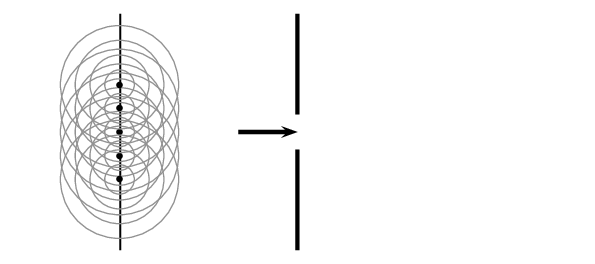Before the the wavefront strikes the barrier the wavefront generates another forward moving wavefront (applying Huygens' principle). Once the barrier blocks most of the wavefront you can see that the forward moving wavefront bends around the slit because the secondary waves they would need to interfere with to create a straight wavefront have been blocked by the barrier.

If you employ Huygens' principle you can see the effect is that the wavefronts are no longer straight lines.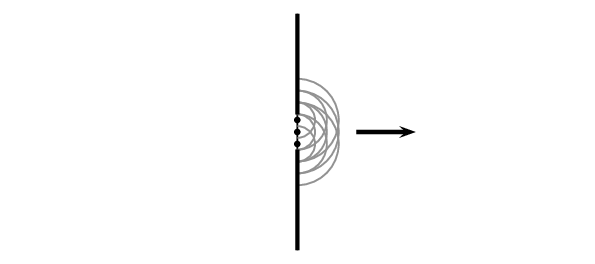### Diffraction patterns (ESBNK)

We can learn even more about what happens after the wavefront strikes the barrier by applying Huygens' principle further.

Each point on the wavefront moving through the slit acts like a point source. We can think about some of the effects of this if we analyse what happens when two point sources are close together and emit wavefronts with the same wavelength and frequency. These two point sources represent the point sources on the two edges of the slit and we can call the source A and source B.

Each point source emits wavefronts from the edge of the slit. In the diagram we show a series of wavefronts emitted from each point source. The black lines show peaks in the waves emitted by the point sources and the gray lines represent troughs. We label the places where constructive interference (peak meets a peak or trough meets a trough) takes place with a solid diamond and places where destructive interference (trough meets a peak) takes place with a hollow diamond. When the wavefronts hit a barrier there will be places on the barrier where constructive interference takes place and places where destructive interference happens.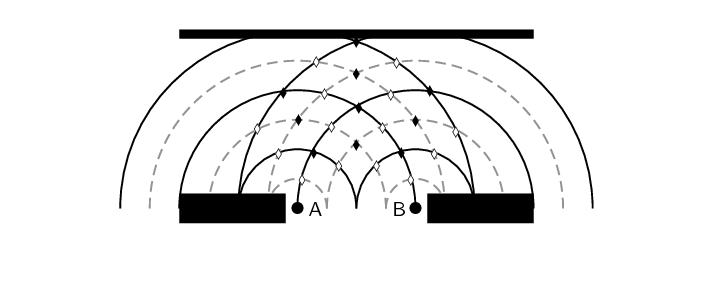The measurable effect of the constructive or destructive interference at a barrier depends on what type of waves we are dealing with. If we were dealing with sound waves, then it would be very noisy at points along the barrier where the constructive interference is taking place and quiet where the destructive interference is taking place.

The pattern of constructive then destructive interference measured some distance away from a single slit is caused because of two properties of waves, diffraction and interference. Sometimes this pattern is called an interference pattern and sometimes it is called a diffraction pattern. Both names are correct and both properties are required for the pattern to be observed. For consistency we will call it a diffraction pattern in for the rest of this book.

The intensity of the diffraction pattern for a single narrow slit looks like this: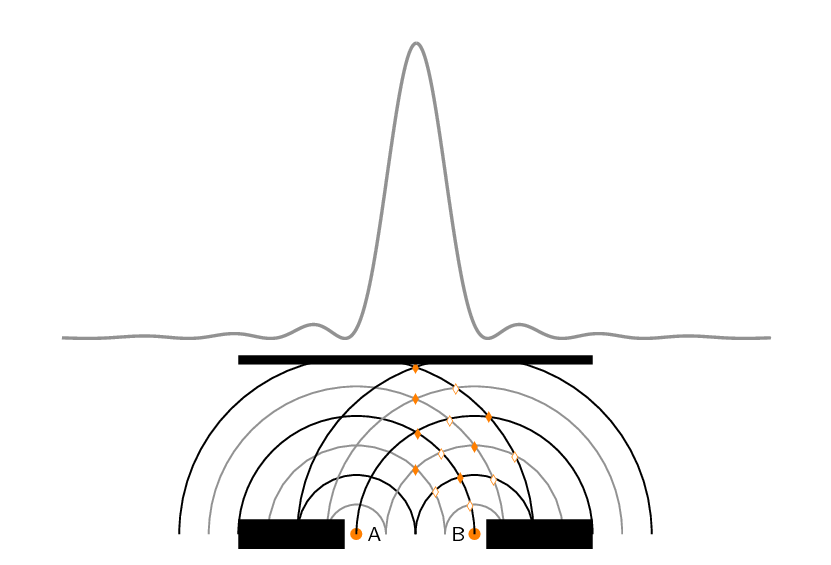The picture above sketches how the wavefronts interfere to form the diffraction pattern. The peaks correspond to places where the waves are adding constructively and the minima are places where destructive interference is taking place. If you look at the picture you can see that if the wavelength (the distance between two consecutive peaks/troughs) of the waves were different the pattern would be different. For example, if the wavelength were halved the sketch would be: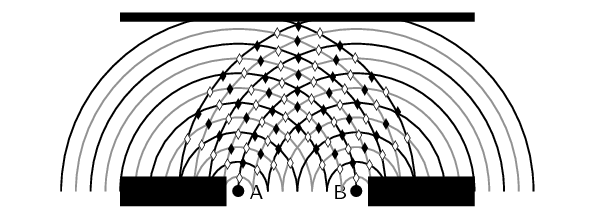The amount that the waves diffract depends on the wavelength. We can compare the spread in the points of constructive and destructive interference by plotting the highlighted points together for the two cases. We have to line up the central maximum from the two cases to see the difference. The case where the wavelength is smaller results in smaller angles between the lines of constructive and destructive interference.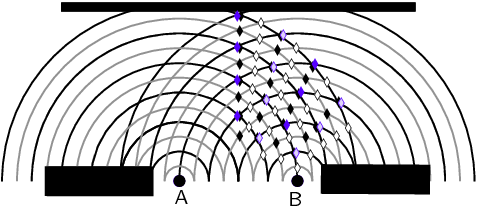It also depends on the width of the slit, changing the width of the slit would change the distance between the points labelled A and B in the sketch. For example, if we repeat the sketch halving the distance between the points A and B we would get: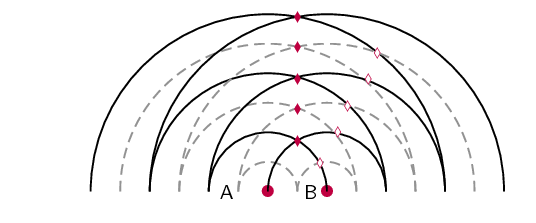We can compare the spread in the points of constructive and destructive interference by plotting the highlighted points together for the two cases. We have to line up the central maximum from the two cases to see the difference. The case where the two points are closer together, in purple, results in bigger angles between the lines of constructive and destructive interference.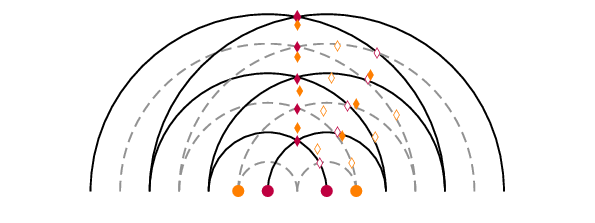temp text

### Effect of slit width and wavelength on diffraction patterns (ESBNM)

Using our sketches we see that the extent to which the diffracted wave passing through the slit spreads out depends on the width of the slit and the wavelength of the waves. The narrower the slit, the more diffraction there is and the shorter the wavelength the less diffraction there is. The degree to which diffraction occurs is:$\text{diffraction}\propto \frac{\lambda}{w}$ where $$\lambda$$ is the wavelength of the wave and $$w$$ is the width of the slit.

We can do a sanity check on the relationship by considering some special cases, very big and very small values for each of the numerator and denominator to see what sort of behaviour we expect (this is not a calculation, just a check to see what sort outcomes we expect when we change wavelength or slit width):

• Set $$\lambda=1$$ and $$w$$ very large, the result will be $$\frac{1}{\text{very big number}}$$ which is a very small number. So for a very big slit there is very little diffraction.
• Set $$\lambda=1$$ and $$w$$ very small, the result will be $$\frac{1}{\text{very small number}}$$ which is a very big number. So for a very small slit there is large diffraction (this makes sense because eventually you are dealing with a point source which emits circular wavefronts).
• Set $$\lambda$$ very large and $$w=1$$, the result will be $$\frac{\text{very big number}}{1}$$ which is a very big number. So for a very big wavelength there is large diffraction.
• Set $$\lambda$$ very small and $$w=1$$, the result will be $$\frac{\text{very small number}}{1}$$ which is a very small number. So for a very small wavelength there is little diffraction.
temp text

### Wave nature of light (ESBNN)

In Grade 10 we learnt about electromagnetic radiation and that visible light is a small part of the EM spectrum. EM radiation is a wave so we should see diffraction for visible light when it strikes a barrier or passes through a slit. In everyday life you don't notice diffraction of light around objects or when light passes through an open door or window. This is because the wavelength of light is very small and the "slits" like doors and windows are quite large.

We can put some everyday numbers into $\text{diffraction}\propto \frac{\lambda}{w}$ to see how much diffraction we expect. White light is combination of light of many different colours and each colour has a different frequency or wavelength. To make things simpler lets just think about one colour, green light has a wavelength of $$\text{532} \times \text{10}^{-\text{9}}$$ $$\text{m}$$. If a wavefront of green light struck the wall of a house with an open door that is $$\text{1}$$ $$\text{m}$$ wide what would we expect to see?

\begin{align*} \text{diffraction} &\propto \frac{\lambda}{w} \\ & \propto \frac{\text{532} \times \text{10}^{-\text{9}}\text{ m}}{\text{1}\text{ m}} \\ &\propto \text{532} \times \text{10}^{-\text{9}} \end{align*}

The result is a very small number so we expect to see very little diffraction. In fact, the effect is so small that we cannot see it with the human eye. We can observe diffraction of green light but for us to get $$\text{diffraction} \propto 1$$ we need the wavelength and slit width to be the same number. So we know the effects of diffraction should become noticeable when the wavelength and slit width are similar. We can't change the wavelength of green light but there are objects called diffraction gratings that have very narrow slits that we can use to study the diffraction of light. We let wavefronts of green light strike a diffraction grating and then put a screen on the other side. We can see where the intensity of the the light on the screen is large and where it is small. For green light on a particular diffraction grating the pattern of green light on the screen looks like:Blue light with a wavelength of $$\text{450} \times \text{10}^{-\text{9}}$$ $$\text{m}$$ and the same diffraction grating will produce:## Worked example 2: Diffraction

Two diffraction patterns are presented, determine which one has the longer wavelength based on the features of the diffraction pattern. The first pattern is for green light:The second pattern is for red light:The same diffraction grating is used in to generate both diffraction patterns.

### Determine what is required

We need to compare the diffraction patterns to extract information about the relative wavelengths so we can decide which one is longer. We know that the diffraction pattern depends on wavelength and slit width through: $\text{diffraction}\propto \frac{\lambda}{w}$

The diffraction grating is the same in both cases so we know that the slit width is fixed.

### Analyse patterns

By eye we can see that the red pattern is wider than the green pattern. There is more diffraction for the red light, this means that: \begin{align*} \text{diffraction}_{red} &> \text{diffraction}_{green}\\ \frac{\lambda_{red}}{w} &> \frac{\lambda_{green}}{w}\\ \lambda_{red} &> \lambda_{green} \end{align*}

The wavelength of the red light is longer than that of the green light.

temp text
Textbook Exercise 6.1

As the width of the slit producing a single-slit diffraction pattern is reduced, how will the diffraction pattern produced change?

More diffraction is observed as the slit width is reduced.

A water break at the entrance to a harbour consists of a rock barrier with a $$\text{50}$$ $$\text{m}$$ wide opening. Ocean waves of $$\text{20}$$ $$\text{m}$$ wavelength approach the opening straight on. Light with a wavelength of $$\text{500} \times \text{10}^{-\text{9}}$$ $$\text{m}$$ strikes a single slit of width $$\text{30} \times \text{10}^{-\text{9}}$$ $$\text{m}$$. Which waves are diffracted to a greater extent?

We need to calculate the diffraction for each type of wave. We start by calculating the diffraction of the water waves:

\begin{align*} \text{diffraction} & \propto \frac{\lambda}{w} \\ & \propto \frac{\text{20}\text{ m}}{\text{50}\text{ m}} \\ & \propto \text{0,4} \end{align*}

The diffraction of the light waves is:

\begin{align*} \text{diffraction} & \propto \frac{\lambda}{w} \\ & \propto \frac{\text{500} \times \text{10}^{-\text{9}}\text{ m}}{\text{30} \times \text{10}^{-\text{9}}\text{ m}} \\ & \propto \text{16,67} \end{align*}

The light waves are diffracted more.

For the diffraction pattern below, sketch what you expect to change if: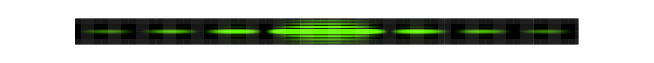the wavelength gets larger

More diffraction would occur. The resulting diffraction pattern is wider: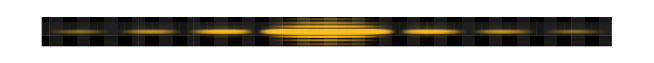the wavelength gets smaller

Less diffraction would occur. The resulting diffraction pattern is narrower: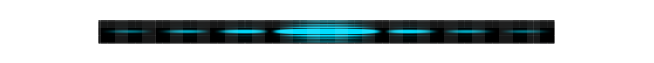the slit width gets larger

Less diffraction would occur. The resulting diffraction pattern is narrower: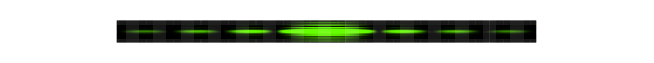the slit width gets smaller

More diffraction would occur. The resulting diffraction pattern is wider: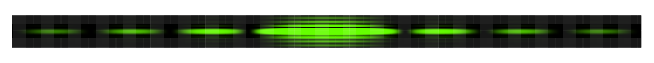the frequency of the wave gets smaller

Frequency is inversely related to wavelength. So the wavelength gets longer and more diffraction would occur. The resulting diffraction pattern is wider:the frequency of the wave gets larger

Frequency is inversely related to wavelength. The wavelength gets shorter and less diffraction would occur. The resulting diffraction pattern is narrower:### Extension: Calculating maxima and minima [NOT IN CAPS] (ESBNP)

There is a formula we can use to determine where the peaks and minima are in the interference spectrum. There will be more than one minimum. There are the same number of minima on either side of the central peak and the distances from the first one on each side are the same to the peak. The distances to the peak from the second minimum on each side is also the same, in fact the two sides are mirror images of each other. We label the first minimum that corresponds to a positive angle from the centre as $$m=1$$ and the first on the other side (a negative angle from the centre) as $$m=-1$$, the second set of minima are labelled $$m=2$$ and $$m=-2$$ etc.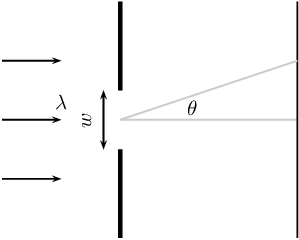The equation for the angle at which the minima occur is given in the definition below:

Interference Minima

The angle at which the minima in the interference spectrum occur is:

$$\sin\theta =\frac{m\lambda }{w}$$

where

θ is the angle to the minimum

w is the width of the slit

λ is the wavelength of the impinging wavefronts

m is the order of the minimum, $$m=±1,±2,±3,...$$

## Worked example 3: Diffraction minimum

A slit with a width of $$\text{2 511}$$ $$\text{nm}$$ has red light of wavelength $$\text{650}$$ $$\text{nm}$$ impinge on it. The diffracted light interferes on a surface. At which angle will the first minimum be?

### Check what you are given

We know that we are dealing with diffraction patterns from the diffraction of light passing through a slit. The slit has a width of $$\text{2 511}$$ $$\text{nm}$$ which is $$\text{2 511} \times \text{10}^{-\text{9}}$$ $$\text{m}$$ and we know that the wavelength of the light is $$\text{650}$$ $$\text{nm}$$ which is $$\text{650} \times \text{10}^{-\text{9}}$$ $$\text{m}$$. We are looking to determine the angle to first minimum so we know that $$m=1$$.

### Applicable principles

We know that there is a relationship between the slit width, wavelength and interference minimum angles:

$$\sin\theta =\frac{m\lambda }{w}$$

We can use this relationship to find the angle to the minimum by substituting what we know and solving for the angle.

### Substitution

\begin{align*} \sin \theta & = \dfrac{\text{650} \times \text{10}^{-\text{9}}\text{ m}}{\text{2 511} \times \text{10}^{-\text{9}}\text{ m}} \\ \sin \theta & = \dfrac{\text{650}}{\text{2 511}} \\ \sin \theta & = \text{0,258861012} \\ \theta & = \sin ^{-1}\text{0,258861012} \\ \theta & = 15 ° \end{align*}

The first minimum is at $$\text{15}$$$$\text{°}$$ from the centre maximum.

## Worked example 4: Diffraction minimum

A slit with a width of $$\text{2 511}$$ $$\text{nm}$$ has green light of wavelength $$\text{532}$$ $$\text{nm}$$ impinge on it. The diffracted light interferes on a surface, at what angle will the first minimum be?

### Check what you are given

We know that we are dealing with diffraction patterns from the diffraction of light passing through a slit. The slit has a width of $$\text{2 511}$$ $$\text{nm}$$ which is $$\text{2 511} \times \text{10}^{-\text{9}}$$ $$\text{m}$$ and we know that the wavelength of the light is $$\text{532}$$ $$\text{nm}$$ which is $$\text{532} \times \text{10}^{-\text{9}}$$ $$\text{m}$$. We are looking to determine the angle to first minimum so we know that $$m=1$$.

### Applicable principles

We know that there is a relationship between the slit width, wavelength and interference minimum angles:

$$\sin\theta =\frac{m\lambda }{w}$$

We can use this relationship to find the angle to the minimum by substituting what we know and solving for the angle.

### Substitution

\begin{align*} \sin \theta & = \dfrac{\text{532} \times \text{10}^{-\text{9}}\text{ m}}{\text{2 511} \times \text{10}^{-\text{9}}\text{ m}} \\ \sin \theta & = \dfrac{\text{532}}{\text{2 511}} \\ \sin \theta & = \text{0,211867782} \\ \theta & = \sin ^{-1}\text{0,211867782} \\ \theta & = 12.2 ° \end{align*}

The first minimum is at $$\text{12,2}$$$$\text{°}$$ from the centre peak.

From the formula $$\sin\theta =\frac{m\lambda }{w}$$ you can see that a smaller wavelength for the same slit results in a smaller angle to the interference minimum. This is something you just saw in the two worked examples. Do a sanity check, go back and see if the answer makes sense. Ask yourself which light had the longer wavelength, which light had the larger angle and what do you expect for longer wavelengths from the formula.

## Worked example 5: Diffraction minimum

A slit has a width which is unknown and has green light of wavelength 532 nm impinge on it. The diffracted light interferes on a surface, and the first minimum is measure at an angle of $$\text{20,77}$$$$\text{°}$$?

### Check what you are given

We know that we are dealing with diffraction patterns from the diffraction of light passing through a slit. We know that the wavelength of the light is $$\text{532}$$ $$\text{nm}$$ which is $$\text{532} \times \text{10}^{-\text{9}}$$ $$\text{m}$$. We know the angle to first minimum so we know that $$m=1$$ and $$\theta =20.77°$$.

### Applicable principles

We know that there is a relationship between the slit width, wavelength and interference minimum angles:

$$\sin\theta =\frac{m\lambda }{w}$$

We can use this relationship to find the width by substituting what we know and solving for the width.

### Substitution

\begin{align*} \sin \theta & = \dfrac{\text{532} \times \text{10}^{-\text{9}}\text{ m}}{w} \\ \sin \text{20,77} ° & = \dfrac{\text{532} \times \text{10}^{-\text{9}}}{w} \\ w &= \dfrac{\text{532} \times \text{10}^{-\text{9}}}{\text{0,3546666667}} \\ w &= \text{1 500} \times \text{10}^{-\text{9}} \\ w &= \text{1 500}\text{ nm} \end{align*}

The slit width is $$\text{1 500}$$ $$\text{nm}$$.